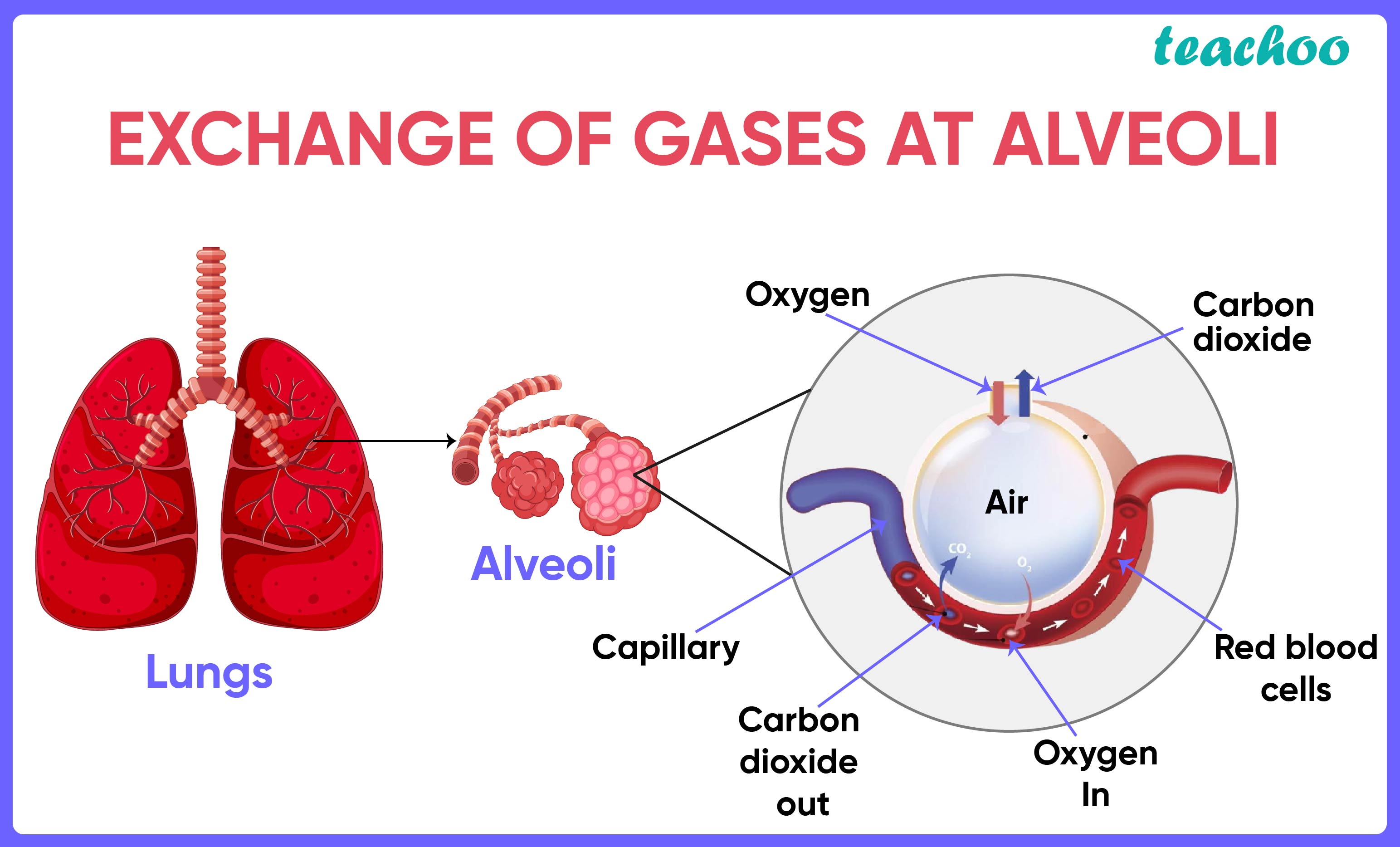Assertion Reasoning Questions (MCQ)

Class 10
Chapter 6 Class 10 - Life Processes (Term 1)

## Reason (R): Alveoli is the site where exchange of  gases occurs.

• The alveoli of lungs are richly supplied with blood
•  Alveoli are the sites where exchange of gases (O 2 and CO 2 ) occurs between blood and the atmosphere.
• The alveoli increase the surface area for this exchange of gases and hence have an extensive network of blood vessels to maximise efficiency.

Hence,

• The assertion is True
• The reason is True

The reason is the correct explanation of the assertionSo, the correct option is (a)